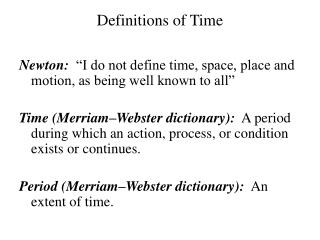DownloadDownload PresentationDefinitions of Time

Definitions of Time

Download PresentationDefinitions of Time

- - - - - - - - - - - - - - - - - - - - - - - - - - - E N D - - - - - - - - - - - - - - - - - - - - - - - - - - -
Presentation Transcript

1. Definitions of Time Newton: “I do not define time, space, place and motion, as being well known to all” Time (Merriam–Webster dictionary): A period during which an action, process, or condition exists or continues. Period (Merriam–Webster dictionary): An extent of time.

2. Definitions of Distance Newton: “I do not define time, space, place and motion, as being well known to all.” Length (MW): a measured distance or dimension Distance (MW): a measure of separation in space Measure (MW): the dimensions . . . of something ascertained by measuring Dimension (MW): measurement of extension (as in length)

3. “God’s Coordinate System” “Absolute, true, and mathematical time . . . flows equably without regard to anything external; relative, apparent, and common time is some sensible and external measure of (time) by the means of motion, which is commonly used instead of true time; such as an hour, day” “Absolute space . . without regard to anything external, remains always similar and immovable. Relative space is some movable dimension or measure of the absolute spaces . . . Absolute and relative space, are the same in figure and magnitude”

4. Evolution of the meter 1 meter = 1 ten–millionth of the distance from the equator to the North Pole Distance between scratches on a bar kept in Serves, France 1,650,763.73 wavelengths of orange light from 86Kr. One inch = 2.54 cm exactly

5. Evolution of the second One second = 1/86,400 of a day Amount of time it takes a pendulum one meter long to swing from one side to the other 9, 192, 631, 770 transitions between the hyperfine levels in 133Cs. (1967) Means one 133Cs vibration is 109 ps (picoseconds)

6. Derived Quantities Speed = distance ÷ time Acceleration = speed ÷ time Force = mass × acceleration (Newtons) Energy = Force × distance

7. Speed of light is special c = 3 × 108 m/s = 186,000 miles/sec (measurement) Define c ≡ 299, 792, 458 m/s exactly One meter is distance light travels in 1/299,792,458 seconds ( = 3 ⅓ ns ) ( = 30.66 133Cs vibrations).

8. Modern Definitions of Standards 1 second = 9, 192, 631, 770 transitions in 133Cs Speed of light = 299, 792, 458 m/s ≈ 3 × 108 m/s. 1 kilgoram is the mass of a standard bar in France 1 meter = distance light moves in ≈ 3⅓ ns. (derived)

9. Other Things We are very familiar with the idea of magnitude: “How Much?” Things like time and mass don’t have direction associated with them: scalars

10. What about Direction? Some quantities require more than just magnitude in order to completely define them. Quantities with both magnitude and direction: vectors. Force is a vector

11. Complications Distance is a scalar; Displacementis a vector. Speed is a scalar; Velocity is a vector; the difference is very important. The magnitude of velocity is speed.

12. Not so complicated Acceleration, Force, Momentum, Angular Momentum: Vectors! Mass, Time, Energy, Power: Scalars!